# Modules, category of

(diff) ← Older revision | Latest revision (diff) | Newer revision → (diff)

The category mod-whose objects are the right unitary modules over an arbitrary associative ringwith identity, and whose morphisms are the homomorphisms of-modules. This category is the most important example of an Abelian category. Moreover, for every small Abelian category there is a full exact imbedding into some category of modules.

If, the ring of integers, then mod-is the category of Abelian groups, and if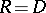is a skew-field, then mod-is the category of vector spaces over.

The properties of mod-reflect a number of important properties of the ring(see Homological classification of rings). Connected with this category is a number of important homological invariants of the ring; in particular, its homological dimension. The centre of mod-(that is, the set of natural transformations of the identity functor of the category) is isomorphic to the centre of.

In ring theory, homological algebra and algebraic-theory, various subcategories of the category of modules are discussed; in particular, the subcategory of finitely-generated projective-modules and the associated-functors (see Algebraic-theory). By analogy with Pontryagin duality, dualities between full subcategories of the category of modules have been studied; in particular between subcategories of finitely-generated modules. For example, it has been established that ifandare Noetherian rings and if there is duality between finitely-generated right-modules and finitely-generated left-modules, then there is a bimodule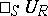such that the given duality is equivalent to the duality defined by the functors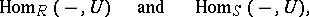the ring of endomorphisms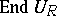is isomorphic to,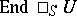is isomorphic to, the bimoduleis a finitely-generated injective cogenerator (both as an-module and an-module), and the ringis semi-perfect (cf. Semi-perfect ring). The most important class of rings, arising in the consideration of duality of modules, is the class of quasi-Frobenius rings (cf. Quasi-Frobenius ring). A left Artinian ringis quasi-Frobenius if and only if the mapping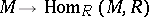defines a duality between the categories of finitely-generated left and right-modules.

How to Cite This Entry:
Modules, category of. Encyclopedia of Mathematics. URL: http://encyclopediaofmath.org/index.php?title=Modules,_category_of&oldid=17739
This article was adapted from an original article by A.V. Mikhalev (originator), which appeared in Encyclopedia of Mathematics - ISBN 1402006098. See original article